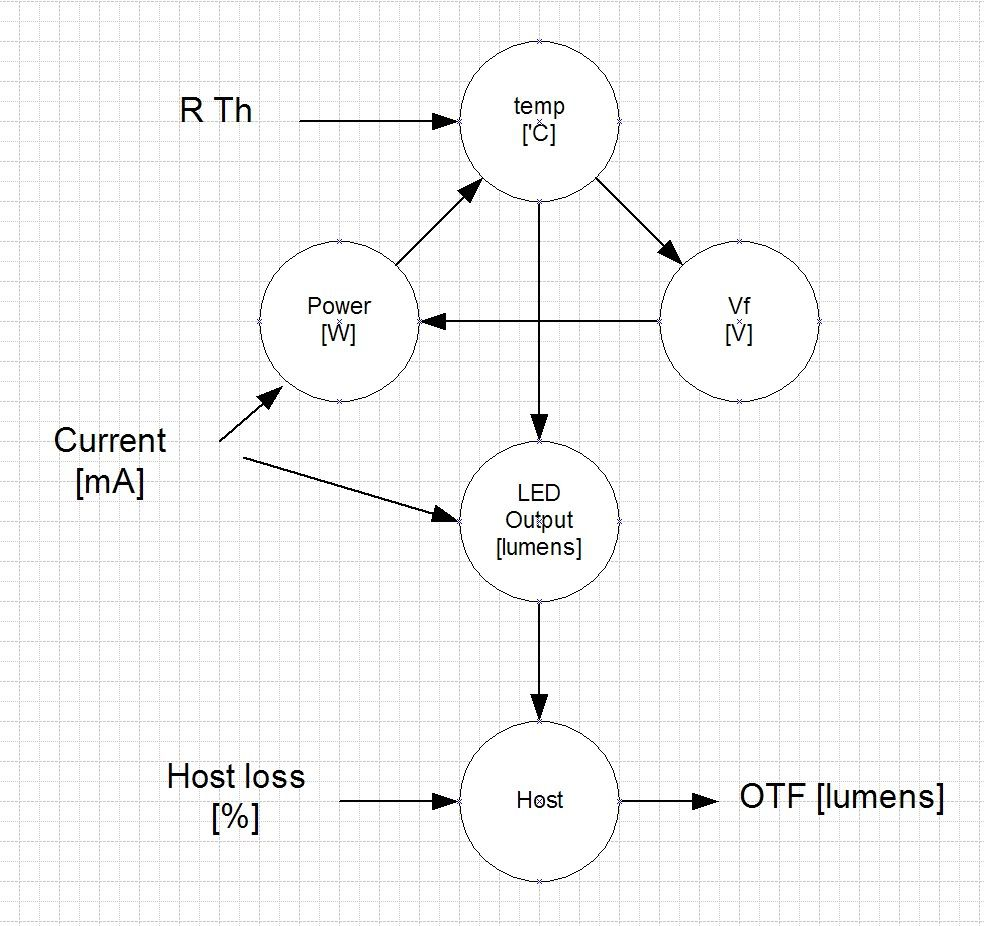# What determines the light output from a flashlight? -use a spreadsheet

1 post / 0 new
sixty545
Offline
Last seen: 4 months 1 week ago
Joined: 10/25/2010 - 14:15
Posts: 702
Location: Denmark (GMT + 1)
What determines the light output from a flashlight? -use a spreadsheet

Frequently the same question pops up: How much light do I get if I use this LED and my driver feeds it with so many mA ?

This is a very relevant question and the answer ought to be: That depends...
We all know thas the light sags when the LED heats up, but how much?
It depends on the construction of the light, how good it is to pass the heat from the LED to the ambient world. The human hand is part of the equation but this is a little beyond my calculation skills.

To calculate the output in lumens is not so simple as multiplying the LED's flux data with the current in mA.
There are a few variables that interact with each other:Current [mA] and V-forward (Vf) of the LED determines the power to the LED.
The Power [W] and the Thermal resistance to ambient (Rth) determines the LED temperature.
(Thermal resistance (Rth) includes all heat transfer resistances in the light:
from LED junction to star to pill to body to ambient).
The temperature influences the LED output and the LED voltage (Vf).
The LED voltage (Vf) determines the power (and now we have the loop responsible for the term "thermal runaway" which can destroy a LED if exposed to a constant voltage).

The current and the LED temperature then determines the LED output.
Finally the host loss determines the OTF lumens number.

None of the variables are linear functions of each other so precise calculations become very complicated.

I made a spreadsheet (Excel) (already published) to give a prediction of output based on current, thermal resistances and host loss. The calculations uses formulas made by curve-fitting to Cree's data sheets. In order to calculate at excess currents I extrapolated the curves to about double the max current. The predictions it gives seems to be pretty accurate in the normal current range.
At least it can be very fun to play with.

Edited by: sixty545 on 05/12/2012 - 03:41